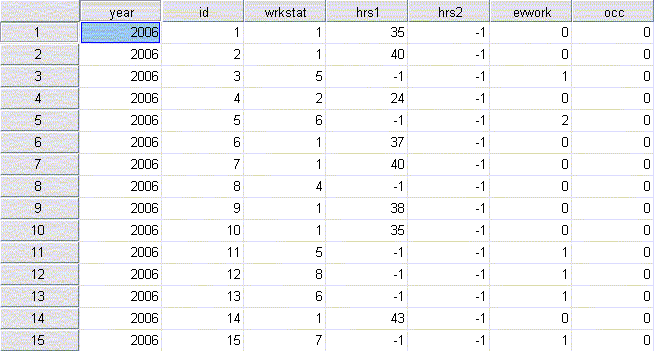# Data Sets and Statistics Resources

## Data vs Statistics

What is the difference between Data and Statistics?

Data is raw information from which statistics are created.
Statistics provides a summary and interpretation of the data.

### Data

• Datasets
• Machine-readable data files, data files for statistical software programs

If you want to understand a phenomenon, you want data. Data can be analyzed and interpreted using statistical procedures to answer “why” or “how.” Data is used to create new information and knowledge.

Raw data is the direct result of research that was conducted as part of a study or survey. It is a primary source. It usually comes in the form of a digital data set that can be analyzed using software such as Excel, SPSS, SAS, and so on. This is what a data set looks like:### Statistics

• Statistical tables, charts, and graphs
• Reported numbers and percentages in an article

If you’re looking for a quick number, you want a statistic. A statistic will answer “how much” or “how many”. A statistic repeats a pre-defined observation about reality.

Statistics are the results of data analysis. It usually comes in the form of a table or chart. This is what a statistical table looks like: